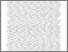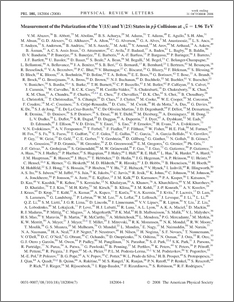# Measurement of the polarization of the $Υ(1S)$ and $Υ(2S)$ states in $p\bar{p}$ collisions at $\sqrt{s}=$1.96 TeV

Collaboration, D0 and M. Abazov, V. and Bertram, Iain (2008) Measurement of the polarization of the $Υ(1S)$ and $Υ(2S)$ states in $p\bar{p}$ collisions at $\sqrt{s}=$1.96 TeV. Physical review letters, 101 (18). ISSN 1079-7114Preview
PDF
e182004.pdf - Published Version

## Abstract

We present a study of the polarization of the $\Upsilon(1S)$ and $\Upsilon(2S)$ states using a $1.3$ fb$^{-1}$ data sample collected by the D0 experiment in 2002--2006 during Run II of the Fermilab Tevatron Collider. We measure the polarization parameter $\alpha=(\sigma_{T}-2\sigma_{L})/(\sigma_{T}+2\sigma_{L})$, where $\sigma_{T}$ and $\sigma_{L}$ are the transversely and longitudinally polarized components of the production cross section, as a function of the transverse momentum ($p_{T}^{\Upsilon}$) for the $\Upsilon(1S)$ and $\Upsilon(2S)$. Significant $p_{T}^{\Upsilon}$-dependent longitudinal polarization is observed for the $\Upsilon(1S)$. A comparison with theoretical models is presented.

Item Type:
Journal Article
Journal or Publication Title:
Physical review letters
© 2008 The American Physical Society
Uncontrolled Keywords:
/dk/atira/pure/researchoutput/libraryofcongress/qc
Subjects:
Departments:
ID Code:
56736
Deposited By:
Deposited On:
07 Aug 2012 09:00
Refereed?:
Yes
Published?:
Published